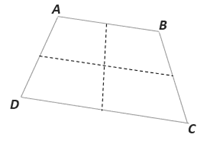Chapter 4.1, Problem 24EElementary Geometry For College St...

7th Edition
Alexander + 2 others
ISBN: 9781337614085

Solutions

Chapter
SectionElementary Geometry For College St...

7th Edition
Alexander + 2 others
ISBN: 9781337614085
Textbook Problem

In quadrilateral ABCD, the midpoints of opposite sides are joined to form two intersecting segments. Try drawing other quadrilaterals and joining opposite midpoints. What can you conclude about these segments in each case?To determine

To conclude:

When the midpoints of the consecutive sides are joined in order.

Explanation

Given:

In quadrilateral ABCD, the midpoints of opposite sides are joined to form two intersecting segments.

Corollary:

A quadrilateral is a four sided closed figure.

From the given quadrilateral ABCD, the midpoints of opposite sides are joined to form two intersecting segments...

Still sussing out bartleby?

Check out a sample textbook solution.

See a sample solution

The Solution to Your Study Problems

Bartleby provides explanations to thousands of textbook problems written by our experts, many with advanced degrees!

Get Started

Find more solutions based on key concepts